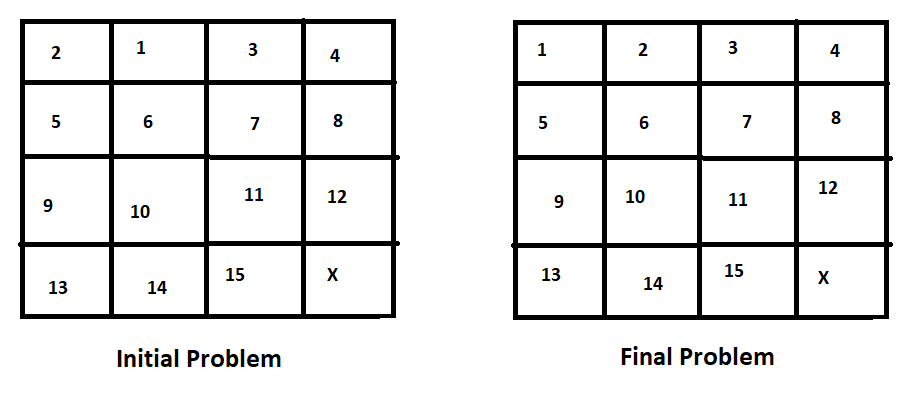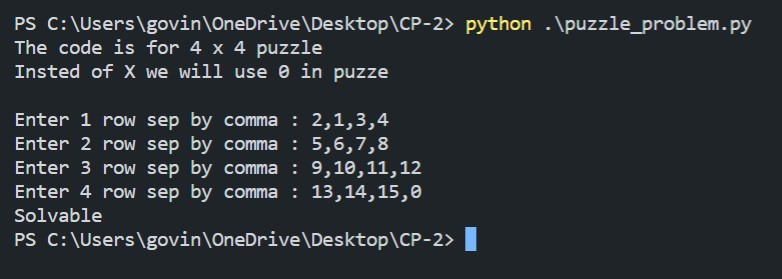## Problem :

Given a 4×4 board with 15 tiles (every tile has one number from 1 to 15) and one empty space. The objective is to place the numbers on tiles in order using the empty space. We can slide four adjacent (left, right, above and below) tiles into the empty space.

For example,## Solution :

```				```
N=4
def inversionCount(arr):
arr1=[]
for y in arr:
for x in y:
arr1.append(x)
arr=arr1
inv_count = 0
for i in range(N * N - 1):
for j in range(i + 1,N * N):
if (arr[j] and arr[i] and arr[i] > arr[j]):
inv_count+=1

return inv_count

def positionOfX(puzzle):
for i in range(N - 1,-1,-1):
for j in range(N - 1,-1,-1):
if (puzzle[i][j] == 0):
return N - i

def isSolvable(puzzle):
invCount = inversionCount(puzzle)

if (N & 1):
return ~(invCount & 1)

else: # grid is even
pos = positionOfX(puzzle)
if (pos & 1):
return ~(invCount & 1)
else:
return invCount & 1

puzzle = []
x = 0
print("The code is for 4 x 4 puzzle")
print("Insted of X we will use 0 in puzze \n")
while(x<=3):
lst = list(map(int,input("Enter "+ str(x+1) +" row sep by comma : ").split(',')))
puzzle.append(lst)
x+=1

# for i in range(x):
#     lst = list(map(int,input("Enter each row sep by comma :").split(',')))
#     puzzle.append(lst)

if isSolvable(puzzle):
print("Solvable")
else:
print("Not Solvable")

```
```

Output :Categories: Informative